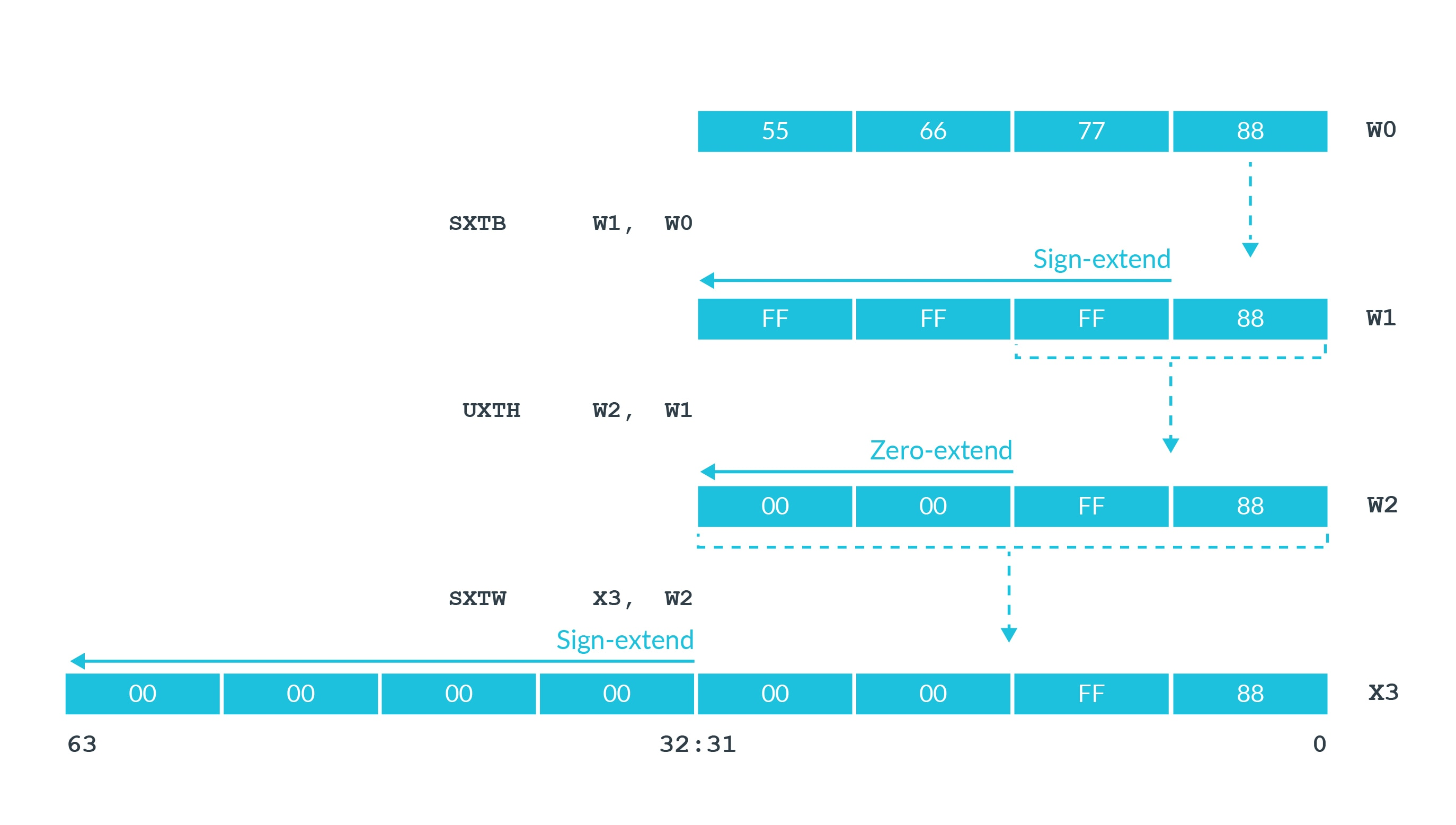## Extension and saturation

Sometimes it is necessary to convert data from one size to another. The `SXTx` (sign extend) and `UXTx` (unsign extend) instructions are available for this conversion. In this conversion, the `x` determines the size of the data being extended, as shown in this figure:In the first instruction, `SXTB`, the `B` means byte. It takes the bottom byte of `W0` and sign extends it to 32 bits.

`UXTH` is an unsigned extension of a halfword (`H`). It takes the bottom 16 bits of `W1` and zero extends it to 32 bits.

The first two examples have `W` registers as a destination, meaning the extension is to 32 bits. The third example has an `X` register, meaning the sign extension is to 64 bits.

##### Sub-register-sized integer data processing

Some instructions perform saturating arithmetic. This means that if the result is larger or smaller than the destination can hold, then the result is set to the largest or smallest value of the destination's integer range.

The data-processing instructions can efficiently handle 32-bit data and 64-bit data. In practice, you often see saturation instructions when handling sub-register calculations. Sub-register calculations are calculations of 16 bits or 8 bits. This table shows some examples of sub-register calculations in C and the generated assembler code:

 C Generated assembler ```uint32_t add32(uint32_t a, uint32_t b) { return a+b; }``` ```add32:  ADD W0,W1,W0  RET``` ```uint16_t uadd16(uint16_t a, uint16_t b) { return a+b; }``` ```uadd16: AND W8,W1,#0xffff ADD W0,W8,W0,UXTH RET``` ```int16_t sadd16(int16_t a, int16_t b) { return a+b; }``` ```sadd16: SXTH W8,W1 ADD W0,W8,W0,SXTH RET```

In the first example in the table, the 32-bit addition maps onto W registers and therefore can be handled easily.

For the 16-bit examples in the table, an extra instruction is necessary. The third example in the table takes the 16-bit inputs, extends them to 32 bits, and then performs the addition. The sequence converts the 16-bit input to 32 bits, using:

`SXTH  W8,W1`

Then, this instruction performs the addition and saturates the result to signed 16 bits:

`ADD   W0,W8,W0,SXTH`

Adding` , SXTH` to the end of the operand list of the `ADD` operation causes the result to use saturating arithmetic. Because the destination is a `W` register, the `ADD` will saturate to a 16-bit integer range.